## 4.16Blended freestream condition

There is a class of problems in CFD that involve external ﬂow around one or more solid bodies, e.g. a vehicle, wind turbine, buildings, etc.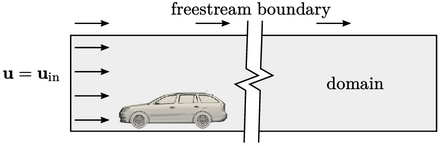A solution domain is speciﬁed which includes the solid body and extends some distance to a free boundary in the far-ﬁeld. A ﬂow velocity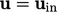is speciﬁed which can be applied as a ﬁxed value type at an inlet patch.

The far-ﬁeld boundary requires attention. The robust conditions at a free boundary for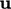and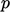are inlet-outlet-velocity and total pressure described in Sec. 4.15 and Sec. 4.7 respectively.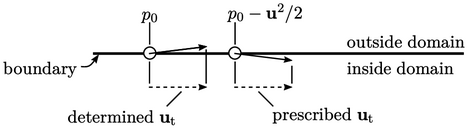The inlet-outlet-velocity requires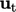to be prescribed for inﬂow which may diﬀer signiﬁcantly from a determined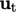when there is outﬂow. Solution accuracy depends on the suitability of the prescribed.

The ﬂow direction in the far-ﬁeld can often be close to tangential to the boundary, especially with a box-shaped domain. If the ﬂow at one face changes from outﬂow to inﬂow,suddenly changes to the prescribed ﬁxed value and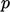decreases by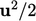. Sometimes a pattern of switching can occur in adjacent faces and repeated switching can slow the convergence of a solution.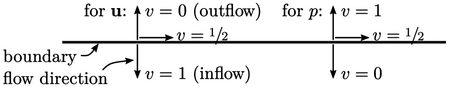The blended freestream condition is a mixed type with zero reference gradient,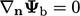, which modiﬁes the value fraction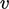as shown above. In the limit that the ﬂow direction is normal to the boundary, the condition becomes the fundamental ﬁxed value and zero gradient types for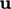and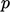.

Between these extremes,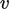is blended linearly, e.g. forby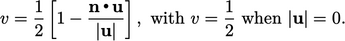(4.27)
This means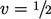for bothandfor tangential ﬂow.

At a boundary face,may be directed normal-inward, causing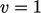by Eq. (4.27 ). The condition can then “lock” at, so to avoid this, the calculation can use a velocity equating to the mean of the face and neighbour cell value, i.e.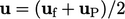.

Note that for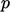, the value fraction is calculated changing the sign of the second term in Eq. (4.27 ) i.e.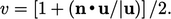The freestream conditions overcome the problem of switching to improve the convergence of solutions. Boundary velocities are determined, not prescribed, which seems to improve accuracy, e.g. in force calculations described in Sec. 8.4 and Sec. 8.6 .

Notes on CFD: General Principles - 4.16 Blended freestream condition